There are all sorts of resonances around us, in the world, in our culture, and in our technology. A tidal resonance causes the 55 foot tides in the Bay of Fundy. Mechanical and acoustical resonances and their control are at the center of practically every musical instrument that ever existed. Even our voices and speech are based on controlling the resonances in our throat and mouth. Technology is also a heavy user of resonance. All clocks, radios, televisions, and gps navigating systems use electronic resonators at their very core. Doctors use magnetic resonance imaging or MRI to sense the resonances in atomic nuclei to map the insides of their patients. In spite of the great diversity of resonators, they all share many common properties. In this blog, we will delve into their various aspects. It is hoped that this will serve both the students and professionals who would like to understand more about resonators. I hope all will enjoy the animations.

Origins of Newton's laws of motion

History of mechanical clocks with animations
Understanding a mechanical clock with animations
includes pendulum, balance wheel, and quartz clocks

## Sunday, July 14, 2013

### 3.20 Derivation of wave properties for transmission linesAll postings by author previous: 3.19 Applying methods of 3.18 to the circuit of 3.17 up: Contents next: 3.21 Reflection and absorption coefficients for transmission lines with loads
 This posting includes flash animations showing the physics discussed. Most computers have a flash player already installed, but if yours does not, download the free Adobe flash player here. Flash animation of Positive and negatively going attenuated waves on transmission lines. Flash animation of Power in attenuated waves on transmission lines..

3.20 Derivation of wave properties for transmission lines

Keywords: transmission line, telegrapher's equation, types of transmission lines, equivalent circuit, wave solution, propagation constant, characteristic impedance, wave energy, wave power, flash animation

Topics covered in this posting

• Transmission lines are widely used in communication technology. We discuss the the various kinds of transmission lines and derive the basic equations for wave propagation on these.
• Much of the behavior of waves on a transmission line is captured by the propagation constant and characteristic impedance of the transmission line.
• We derive equations for these.
• We discuss energy and power of the waves on transmission lines.

1. Derivation of telegrapher's equationsFig. 1. Various types of transmission lines. All are characterized by two conductors separated by an insulator and having uniform (or approximately uniform) cross sections. Coaxial cable see the biggest use in cable TV distribution. Twisted pair is used for telephone and DSL. The pair of insulated wires are twisted together (twist not shown here) and usually enclosed in a cable with many other twisted pairs. Twin line was used for many years to route TV signals from roof top antennas to TV's. Many microwave devices are built on a plane insulating substrate that has a conducting ground plane on the bottom side. Much of the math of this posting was started by Oliver Heaviside in an effort to understand the wave effects occurring on long distance telegraph lines in the 1880's.

This posting is meant to be a summary of the rationale behind the wave equations for transmission lines. It also includes discussions of some of the properties of the waves based on the wave equation.

A transmission line consists of a pair of conductors that are uniform in cross section over an extended length and separated by a likewise uniform insulator. Fig. 1 shows several standard transmission lines. More are discussed in this reference and here. Also, much of the equations for transmission lines can be applied, with some restrictions, to plane wave propagation through a uniform medium and to wave propagation through waveguides and optical fibers. In this posting we will focus on waves in traditional transmission lines.

The standard transmission line can be electrically modeled by the circuit shown in Fig. 2, where the L-R-C-G unit cell is repeated many, many times down the length of the transmission line. We consider the case where the unit cell is very short compared to the wavelengths we will investigate. That is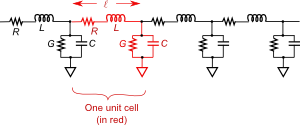Fig. 2. Equivalent circuit of a lumped-parameter transmission line in the limit that ℓ is very small compared with a wavelength.

<< λ    .    (1)

We now write two differential equations governing the voltage and current along the transmission line:

where ΔV is the voltage drop across one unit cell of the circuit in Fig. 2, i.e. across an L R combination of a cell. The current I of a cell is the current passing through L and R of a cell. The change in current ΔI  is the change in current from one unit cell to the next due to current being drained off by the conductance G between the conductor and ground and by the capacitor if the voltage across it is changing, i.e. it is being charged or discharged.

For the component values L, R, C, and G, we substitute the values per unit length for the transmission line times the length of a cell. That is, for L we substitute L1, the inductance per length times the length of our unit cell. Using component values per unit length makes more sense for the uniform pair of conductors. The equations for the component values per unit length for some standard transmission lines can be derived using standard electromagnetic theory (see this reference - towards its end). Note that R1 is the resistance per unit length of the conductors and G1 is the leakage conductance between the conductors (also per unit length). Both R1 and G1 cause signal loss (attenuation) in real transmission lines. Sluggishness in dielectric response [2nd ref] of the electrical insulators in transmission lines is also a common cause of loss, but can be considered a frequency dependent component of G1 for the sake of calculation.

We change into Δx and divide both equations of (3) by Δx:

Finally, we take the limit of very small ℓ's, i.e. very small Δx's, in which the Δ's are replaced by partial differentials:

The equations in (5) are known as the telegrapher's equations and were developed by Oliver Heaviside in the 1880's to describe the electrical properties of long distance telegraph lines which were the developing new technology of the day.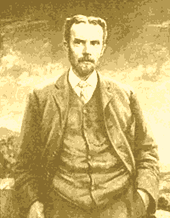The two equations in (5) can be combined to eliminate I and yield a wave equation:.    (6)

With some rearrangement, this can also be expressed as:

2. Lossless solutions to the wave equationIf the loss elements, R1 and G1, are both zero, then this equation becomes simple:

which has solutions of the form:

where A and B are complex constants representing the complex amplitude (amplitude and phase shift) of the positive and negative going sinusoidal traveling waves.

ω is the angular frequency (radians per second) of the wave and κ is the wavenumber (radians per meter). They are related to the velocity c of the wave by:

We can also solve for the characteristic impedance of the transmission line. The characteristic impedance is defined as the ratio of the voltage in a traveling wave propagating in only one direction to the current in the same wave. We start with the left equation of (5) with R1 = 0  and substitute either the first or second term (either the positive or negative going traveling wave) of (8) into it:where we have also assumed that the current I  has the same form as does the voltage, i.e. I = Aei(ωt ∓ κx) . The minus sign in (10) is to be used for positive going traveling waves while the plus sign is for negative going traveling waves.

Using (9) along with (10) we can calculate the characteristic impedance Z0:

where the + sign is used for positive going traveling waves and the minus sign for negatively going traveling waves.

For more about simple waves like this, see an earlier posting.

3. Wave solutions with loss

If R1 and/or G1 is not zero, then the equations are messier and it is useful to start off assuming a sinusoidal solution. Conventionally one picks a general solution of the form:where γ is the propagation constant. Note that there is no complex constant i before the γ in the exponent of (12).

We next substitute (12) into (6) and cancel the factors common to all terms. This yields:

This can be rearranged and solved for γ :

where α and β are defined to be the real and imaginary parts of the propagation constant γ . We can understand the roles α and β play by substituting the first part of (14) back into (12):

We see from the last part of (15) that α occurs in the factor e−αx which modifies the amplitude A making the effective amplitude of the wave become smaller as it propagates. For this reason α is known as the attenuation constant (units are 1/meters or nepers/m where neper is a "dimensionless unit", similar to radians). The second constant β serves the same role as did the wavenumber κ in (8) and governs the wavelength, i.e. λ = 2π/β . β is known as the phase constant with units of radians per meter.

Note that the ± sign in front of the square root in (14) allows for both positive or negative going waves. The + sign is used with positive going waves and makes (15) have positive values of α and β. This results in the waves being attenuated more and more for larger x value as we would expect and also results in sinusoidal functions that move in the direction of positive x as time increases (with attenuation). When we use the minus sign in (14) we get negative values of α and β which cause more attenuation as one goes in the negative x direction, and the sinusoids will move in the negative x direction as time progresses.

Various waves with attenuation as given by Equation (15).
Fig. 3a. Positive going traveling wave with attenuation. Mouse over the animation to activate it. Click on it to restart it.
Fig. 3b. Negative going traveling wave with attenuation.
Fig. 3c. Mix of positive (red) and negative (green) going traveling waves created by a wave hitting a partially absorbing barrier on the right side. The absorption coefficient is smaller than in the above two animations. This animation shows the complicated nature of the mix. The total wave is shown in blue.

To calculate the characteristic impedance as we did in section 2 above, we substitute (12) into the first equation of (5):

We solve for V / I  to give us: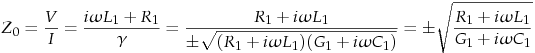.    (17)

Again we see the ± sign in the equation. Because the ± sign came from (14) we interpret its use as we did for (14). That is, the plus sign is to be used for positive going waves while the minus sign is for negative going waves.

4. Allowed ranges of the arguments of the propagation constant and characteristic impedance

We next investigate the allowed ranges of the complex arguments of γ and Z0 . We rewrite (14) and (17) in terms of the series impedance elements and the parallel admittance elements (for positive going waves):where we define the series impedance and parallel admittance as:

Because all four of the component values (R1, L1, G1, and C1) are real and positive (except in really odd environments like an active gain area of a laser), the values of Zs and Yp are restricted to the first quadrant of the complex plane, i.e. positive real and imaginary parts. That is to say that if we express Zs and Yp in polar form:,    (20)

then the arguments of both Zs and Yp will be in the range of 0 to π/2 radians.

Then (18) means that the arguments in (20) will be given by:.    (21)

Equation (21) indicates that the argument of γ is the average of the arguments of the series impedance and the parallel admittance. The argument of Z0 is half the difference between the arguments of the series impedance and the parallel admittance. This means that arg(γ)  being an average will be in the range of 0 to π/2 radians, while arg(Z0) will be in the range of −π/4 to +π/4 . Below in Fig. 4 we show these ranges graphically.

Negative going waves have a minus sign in front of both equations in (18). This will have the effect of inverting both γ and Z0. This will mean that for negative going waves arg(γ) will range from −π to −π/2 radians while arg(Z0) will range from 3π/4 to −3π/4 radians (centered around π radians).

Fig. 4. Allowed ranges of the arguments of the propagation constant and characteristic impedance
Typical complex values of γ and Z0 Ranges of the complex propagation constant γ Ranges of the complex characteristic impedance Z0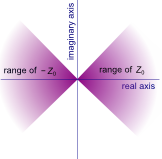Fig. 4a. Complex vectors of typical values used in (18) and (21).Fig. 4b. Possible ranges of the propagation constant γ for real positive component values of R, L, G and C. Positive going traveling waves have propagation constants in the first quadrant while negative going waves have propagation constants in the third quadrant. Regions of heavy attenuation (large α) and lossless propagation (small γ) are also indicated.Fig. 4c. Possible ranges of the complex propagation constant Z0 for real positive component values of R, L, G and C. The range in the positive x direction is for positive going traveling waves. The range in the negative x direction is for negative going traveling waves.

The argument (complex angle) of Z0 does not correlate with losses, except to say that a lossless wave medium will have an argument of Z0 equal to zero, i.e. its complex vector will be horizontal and Z0 will be entirely real. On the other hand, zero argument does not necessarily indicate lossless wave propagation. In fact if a lossy line meets the Heaviside or distortionless conditionG1C1 = R1L1 ) it will have a totally real characteristic impedance even though it has loss.

The argument (complex angle) of Z0 indicates the phase difference between the voltage and current components of a wave.

The heavily attenuated electromagnetic wave propagation into a metal has a propagation constant with an argument of π/4 and an impedance with an argument of π/4 also.

5. Energy and power in waves

In a transmission line the energy in a wave is stored in both the inductance and capacitance of the line. The total energy stored in both per unit length of the transmission line is given by:

for real functions (sinusoidal) of the voltage V and current I. For complex forms of the voltage and current, we use:.    (23)

We cannot use |V|2 or VV* where V* is the complex conjugate of V because that would give the time averaged power. We want the time varying power. This issue is discussed in this posting.

The power transmitted along the line is given by:,    (24)where the first form above is appropriate for real (sinusoidal) forms of I and V.

The power dissipated per unit length by the transmission line is given by:where the first form above is appropriate for real sinusoidal forms of I and V.

With all the equations (22) - (25) above we could use the relation between I and V expressed in equation (11) to eliminate one of these two variables, if the wave was entirely a traveling wave, entirely a standing wave, or a known constant fraction of the traveling waves in each direction.

Below we plot the above energy and powers for various waveforms. The first set of graphs (Fig. 5) is for pure attenuated traveling waves. The second set is animated (mouse over each) and shows these quantities when there is a standing wave added to the traveling wave.

Various attenuated waveforms with energy and power along a transmission line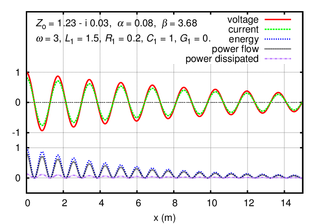Fig. 5. Each of these three graphs shows the voltage V, current I , energy (in the capacitance and inductance of the transmission line), power flow along the transmission line, and power dissipated by the resistance and leakage conductance along the transmission line. All of these are shown versus distance x along the transmission line. These all represent a snap shot in time. In all cases the waves are moving to the right and all these parameters will move to the right with time, shrinking in amplitude as they move to the right.

The graph directly above shows the no-attenuation case, where R1 = 0 and G1 = 0 .The other two cases show varying amounts of attenuation, both with R1 > 0 and G1 = 0. Note that even with the asymmetry in R1 and G1 the phase of I compared with V is hardly noticeable. The message is that it takes extreme attenuation to see a noticeable phase shift between I  and V .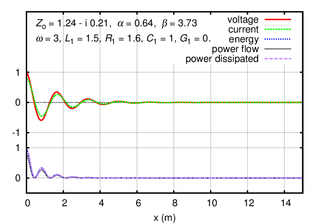The animated graphs below show the complication of a mix of traveling and standing waves. In Fig. 6 we show cases where traveling waves with various attenuations hit a wall and reflect. We will see that depending on the strength of the reflected wave, the energy and power flow is complicated. We will visit this complication mathematically at the end of the next posting.

Reflection of waves with various attenuations showing energy
Fig. 6a. Traveling wave with heavy attenuation. Once the wave hits the right edge, it reflects and makes a partial standing wave. The upper traces are the voltage (red) and current (purple). The lower traces are energy in the wave (green), power being transmitted (blue), and dissipated power (yellow). The various energies slide to the right along with the wave. Note that all the lower curves (energy curves) have twice the spatial frequency compared with the upper curves. Also the lower curves are always positive. Mouse over the animation to activate it. Click on it to restart it. Parameters used: R1 = 0.1ohm/m, L1 = 0.2H/m, C1 = 0.1F/m, and G1 = 0.
Fig. 6b. Traveling wave with medium attenuation. Once the wave hits the right edge, it reflects and sets up a complicated traveling/standing wave mix. You can observe this type of behavior watching a water wave run into the wall of a pier and the reflected wave interacts with the oncoming waves. Note that the transmitted wave (blue) can be negative as well as positive when the reflected wave interacts with the incident wave. Power that cyclically switches direction (or sign) is called reactive power. Parameters used: R1 = 0.003ohm/m, L1 = 0.2H/m, C1 = 0.1F/m, and G1 = 0.
Fig. 6c. Traveling wave with no attenuation. Once the wave hits the right edge, it reflects, setting up a pure standing wave. Note that in the standing wave, the current and voltage are 90 degrees out of phase in both the time and distance domains. Note also that the energy (green) in the wave shifts position back and forth. The dissipated power is zero because this is the zero attenuated case. Parameters used: R1 = 0, L1 = 0.2H/m, C1 = 0.1F/m, and G1 = 0.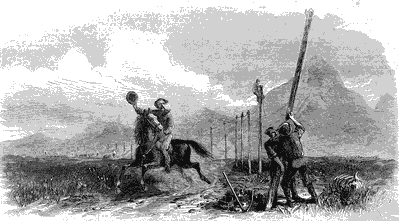Painting by George M. Ottinger. Displacement of the pony express by the telegraph.
 All postings by author previous: 3.19 Applying methods of 3.18 to the circuit of 3.17 up: Contents next: 3.21 Reflection and absorption coefficients for transmission lines with loads